Garrett Mayock's Blog

# Holt's α,β Model for Predicting Time Series with Trends

### Following along Managing Supply Chain Operations Chapter 2 using Python, cont'd.Garrett Mayock posted 2019-03-01 05:58:28 UTC

As before: I am reading Managing Supply Chain Operations. Chapter 2 details Forecasting and Demand Management methods. I figured I'd kill two birds with one stone (learning the material and learning Python) by recreating the chapter material in Python. I anticipate answering the chapter exercises in Python as well, but will save that for another blog post. (Writer's note - please look at the other Chapter 2 blogs (naive, non-naive, linear regression). Click here to download the Excel file I ingested.

Forecasting trending data with Holt's trend (α,β) model

Holt's trend model uses additional smoothing factors beyond simple linear regression. These factors - α and β - represent the data smoothing factor and the trend smoothing factor. Both must be between 0 and 1. Holt's model is a type of exponential smoothing model that corrects for trends.

Holt's model, however, is dependent upon linear regression to determine the initial "base level" (intercept, called S0) and "growth trend" (slope, called G0). Then, as time goes on, Holt's model can be used to update the forecasts without "overreacting" (it is, after all, a type of exponential smoothing model).

Now, you can think of α and β as how much "new information" you update St and Gt when new information (Dt) comes in. Let's say you have demand information for the past 12 months. A least-squares regression analysis will show an intercept of 12,341 and a slope of 84 for the line of best-fit: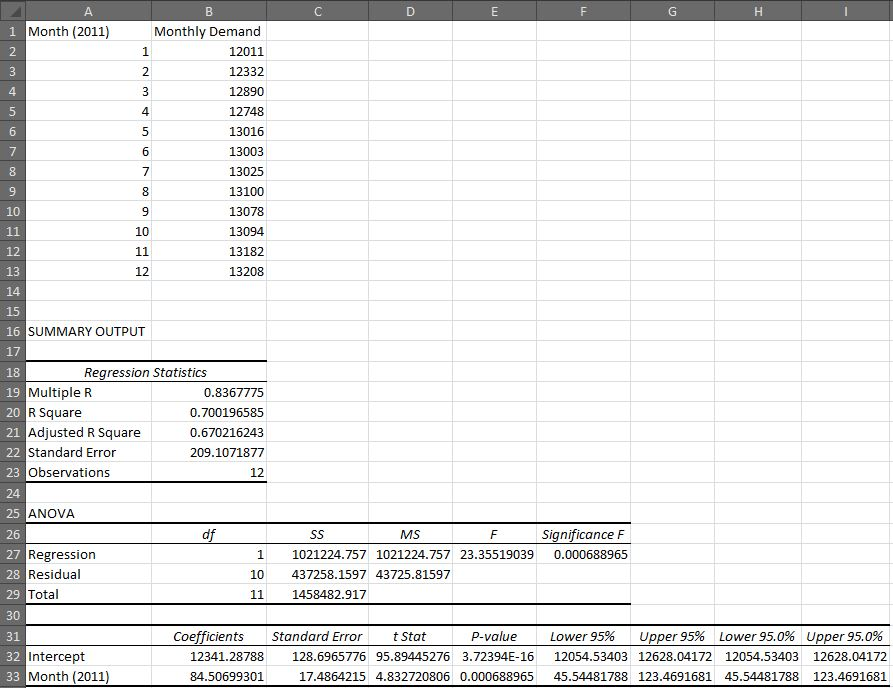If we are using that information to forecast the next 12 months, we would reset the base to 13,208 (most recent demand) and add one period's growth to get January's forecast, two periods' for February's, and so on, resulting in 13,293, 13,377, and 13,462 for the first three months of the new year.

Say, however, that January's demand came in low at 12,600. If you were to follow the same procedure as before - recalculating the regression and setting the base to the most-recent month - it would result in a massive change in the forecast (and a much lower R-squared score), with a new base of 12,600 and a new growth of 57: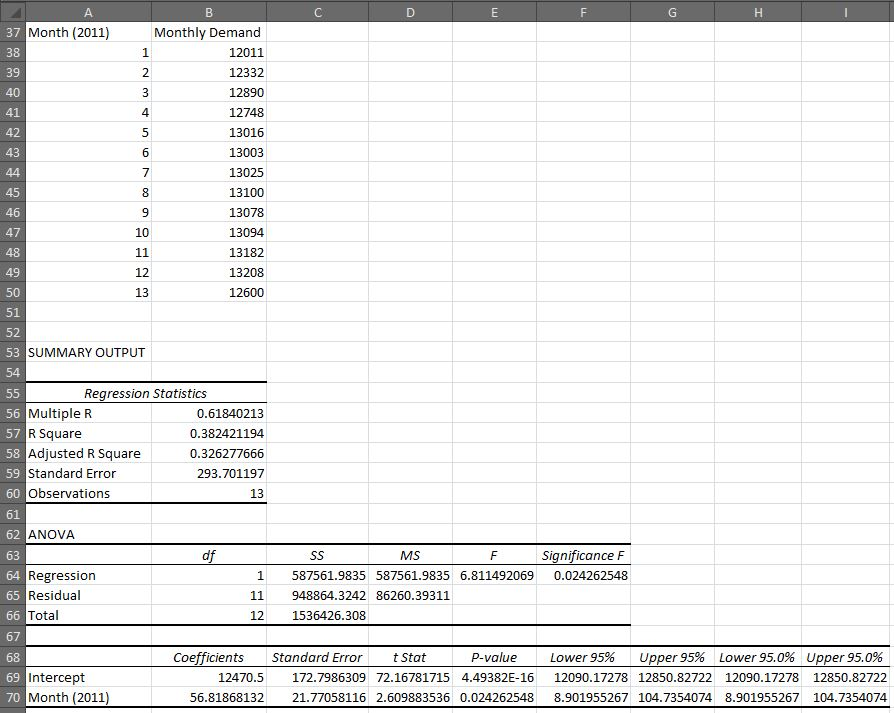Using Holt's trend model, however - with an arbitrary α of 0.1 and β of 0.2 - results in a new base of 13,223 and a new growth of 71. That's because only 10% (α) of the new information for the base is being taken into account, and only 20% (β) for the slope.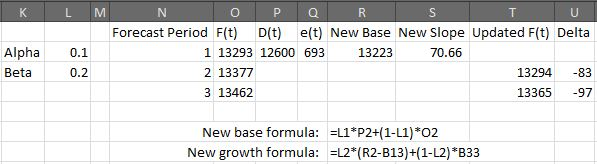Now, we can plot how these lines look, to compare the different forecasts. The code to do it is:

```df = pd.read_excel('filepath/chapter_2_instruction.xlsx', sheet_name='2.4_figure_2.15', header=0, index_col=None, nrows=24, usecols=[23,24,25,26,27,28])

plt.ylabel('Widget Demand (000s)');
plt.axes().yaxis.grid(linestyle=':');
plt.xlabel('Month');
plt.xlim(0,25);
plt.title('Widget Demand Data');
plt.plot(df['Month'], df['Monthly Demand'], marker='D', color='red');
plt.plot(df['Month'], df['Initial Forecast'], marker='o', color='orange');
plt.plot(df['Month'], df['Updated Regression'], marker='o', color='green');
plt.plot(df['Month'], df['Updated Using Holt\'s'], marker='o', color='blue');
plt.legend();
plt.show();
```

Note how I use \ to escape the ' in the column name with the word "Holt's". This is so that Python doesn't interpret that ' as the end of the string.

Look at the graph: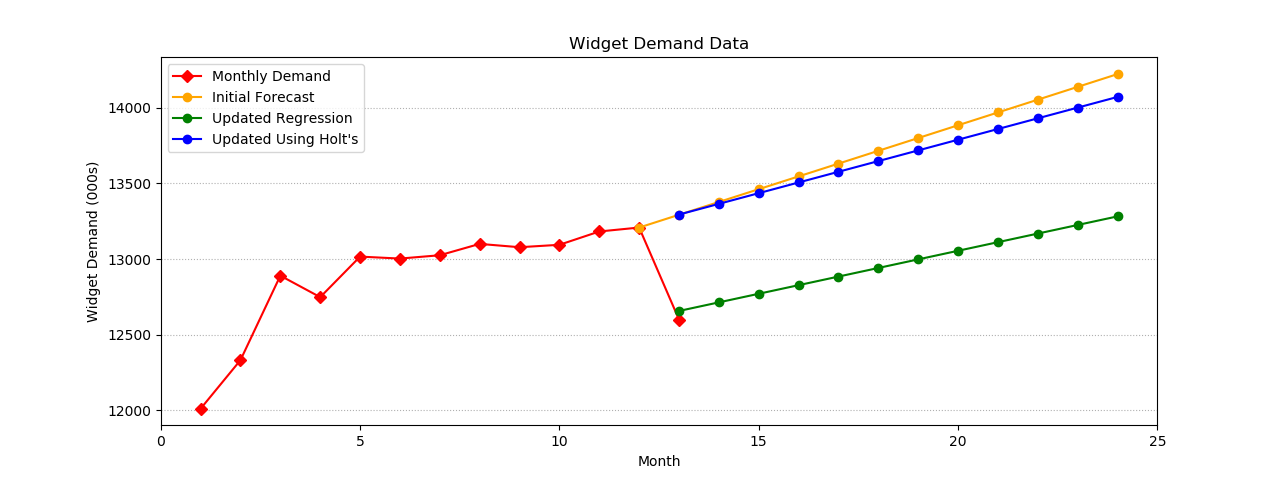You can see that Holt's model is much less affected by the new information than the other method.

Optimizing α and β using Excel

Holt's model's α and β parameters can be optimized using Microsoft Excel Solver. Take a look: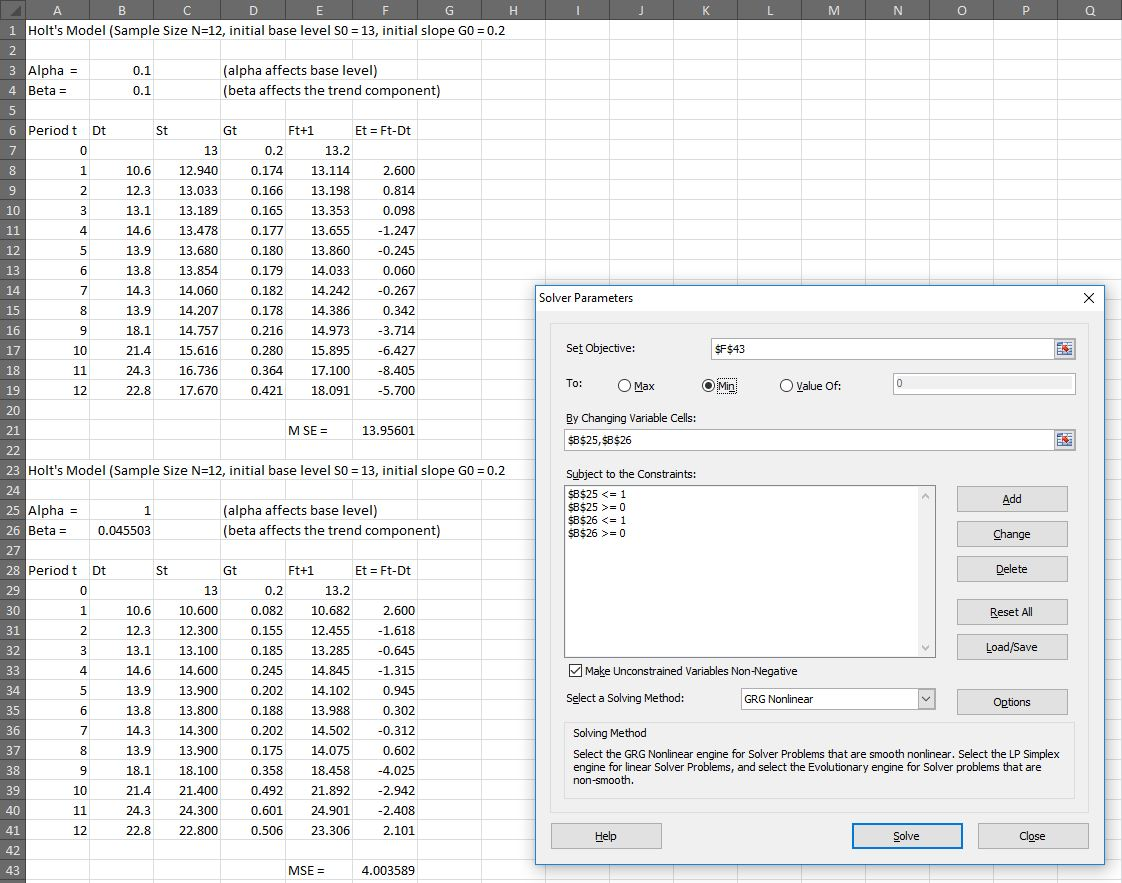Now, the book didn't do the following, but for comparison and learning's sake, let's use Microsoft Excel's Analysis ToolPak add-in to perform a least-squares regression on the data in the above screenshot: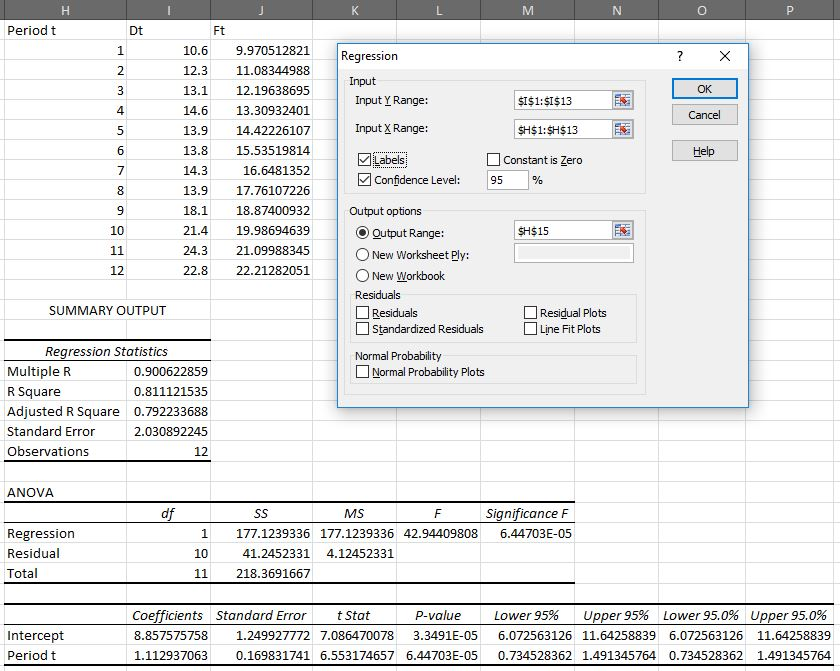And now let's plot the optimized Holt's model's predictions and the predictions from regression analysis side by side. I can either add the linear regression's forecast to the Excel (you'll see I've done that in column J if you download the file) or use Python to populate it. I'll do both:

```# This ingests the optimized Holt's table - period, demand, and forecast

# This drops the extra row we have in that table in the Excel file
df_holts = df_holts[1:]

# This shifts the Ft+1 column down one, and renames it Ft, so the forecast lines up with the actual period it forecasts
df_holts['Ft+1'] = df_holts['Ft+1'].shift(1)
df_holts_map = {'Ft+1':'Ft'}
df_holts = df_holts.rename(columns=df_holts_map)

# This ingests the linear regression table - period, demand, and calculated forecast

# This merges the two dataframes into one
df = pd.merge(df_holts, df_linreg, how='outer', on=['Period t','Dt'])

# This code is cosmetic. It renames the columns first to numbers 0-3 using df.columns, then creates and uses a dictionary to use df.rename()
df.columns = ['0','1','2','3']
df_map = {'0':'Period','1':'Demand','2':'Holt\'s forecast','3':'Excel regression forecast'}
df = df.rename(columns=df_map)

# This code trains a regression model and creates a column with the models predictions, to show that it comes out the same as the Excel regression forecast
import statsmodels.api as sm
results = sm.OLS(df['Demand'], X).fit()
df['sm.OLS regression forecast'] = pd.DataFrame(results.predict(X))

plt.ylabel('Widget Demand (000s)');
plt.axes().yaxis.grid(linestyle=':');
plt.xlabel('Month');
plt.title('Widget Demand Data');
plt.plot(df['Period'], df['Demand'], marker='D', color='red');
plt.plot(df['Period'], df['Holt\'s forecast'], marker='.', color='blue');
plt.plot(df['Period'], df['Excel regression forecast'], marker='o', color='orange');
plt.plot(df['Period'], df['sm.OLS regression forecast'], linestyle='--', marker=None, color='black');
plt.legend();
plt.show();
```

Which creates: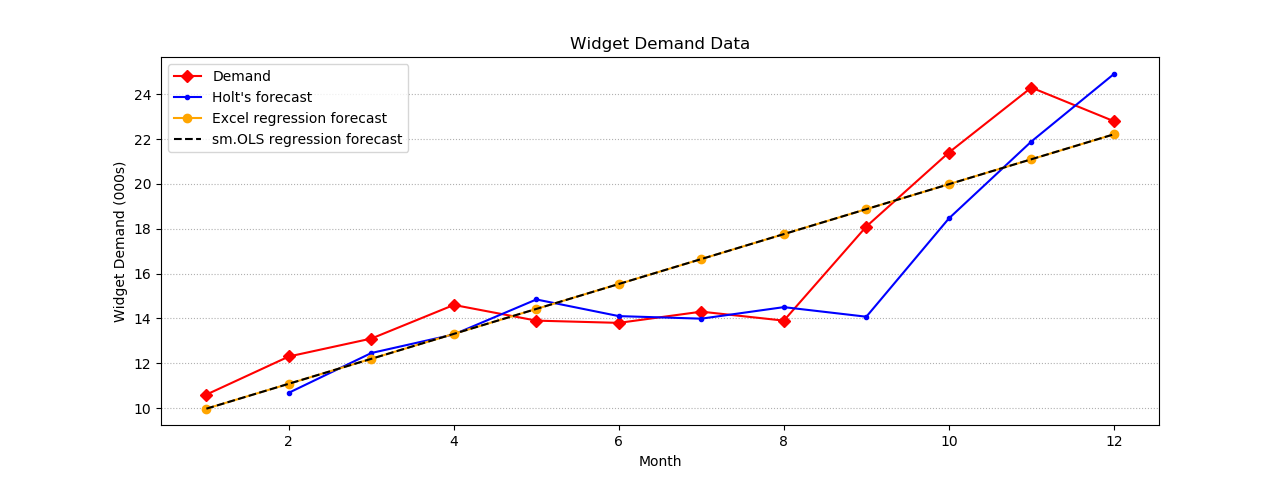Note how the two regression lines sit on top of each other - that's because they are the same type of regression on the same data, just one being calculated in Excel and the other in Python. This is done simply to show that both methods yield the same result.

We can even plot the forecasts out in the future. Remember Holt's model has a new intercept and slope calculated every period - we can use the most recent period's intercept and slope to calculate out an arbitrary number of periods in the future, and we can do the same with either regression model's function. The ingestion process will change a bit, as we're going to use Python to grab Holt's slope and intercept from Excel, and the OLS model code will change a bit too:

```# This ingests slightly more data than before, by including the St and Gt columns

# This drops the same row, shifts demand, renames the columns, and resets the index as before
df = df[1:]
df['Ft+1'] = df['Ft+1'].shift(1)
df_map = {'Period t':'Period','Dt':'Demand','Ft+1':'Holt\'s forecast'}
df = df.rename(columns=df_map)
df = df.reset_index(drop=True)

# This grabs the important values from the extra columns we ingested - the new base and new growth - then drops the columns
holts_base = df['St']
holts_growth = df['Gt']
df = df.drop(columns=['St','Gt'])

# This creates a dataframe with new periods ranging from one above the previous max to twelve beyond that
new_periods = pd.DataFrame([i for i in range (max(df['Period'])+1, max(df['Period'])+1+12)],columns=['Period'])

# This defines a function that we will be using to create new predictions using Holt's model
def holtsPrediction(period):
ypred = holts_base + holts_growth*(period-12)
return ypred

# This actual creates those new predictions
new_periods['Holt\'s forecast'] = [holtsPrediction(i) for i in range( min(new_periods['Period']), max(new_periods['Period'])+1 )]

# This merges the new data on the first dataframe we created
df = pd.merge(df, new_periods, how='outer', on=['Period','Holt\'s forecast'])

# This creates the OLS predictions for all periods using StatsModels - note how we train it only on periods we have data for
import statsmodels.api as sm
results = sm.OLS(df['Demand'][0:12], X).fit()
df['sm.OLS regression forecast'] = pd.DataFrame(results.predict(Xfull))

# This plots it as before
plt.ylabel('Widget Demand (000s)');
plt.axes().yaxis.grid(linestyle=':');
plt.xlabel('Month');
plt.title('Widget Demand Data');
plt.plot(df['Period'], df['Demand'], marker='D', color='red');
plt.plot(df['Period'], df['Holt\'s forecast'], marker='.', color='blue');
plt.plot(df['Period'], df['sm.OLS regression forecast'], linestyle='--', marker=None, color='black');
plt.legend();
plt.show();
```

And the result: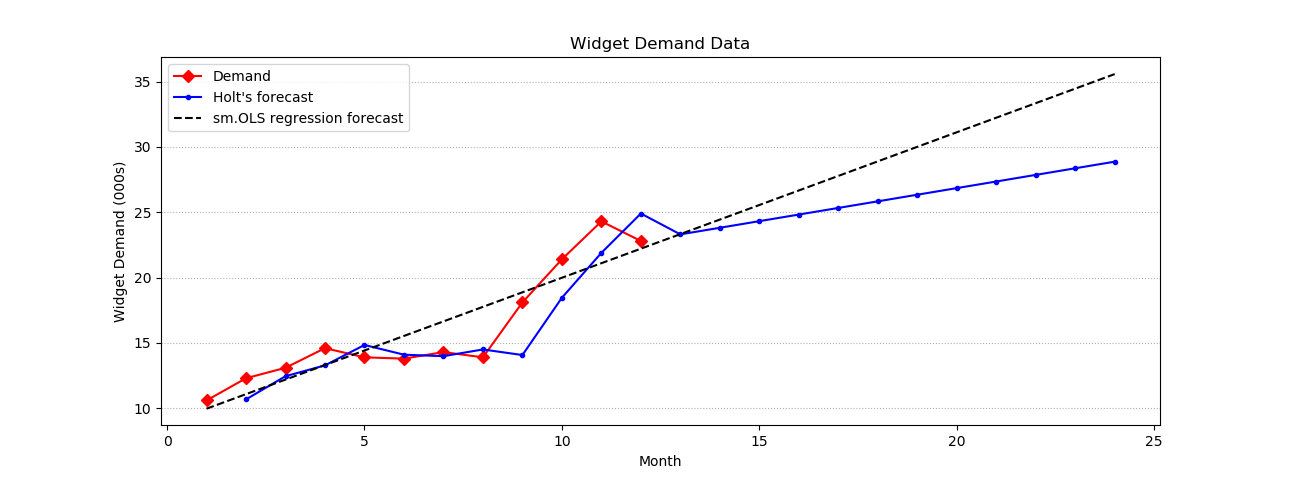Finding a reason to use Holt's

Sadly, I don't think the above use case, as provided to us in the textbook, was really all that useful for understanding the virtue of Holt's model. Some quick internet sleuthing didn't reveal any "aha!" realization of this, either, as most materials online seem to be heavily technical and don't really explain why Holt's may be more applicable in one situation than another.

I'm going to try one more thing with the data we looked at first - but rather than looking only from period 13 on, I will plot the lines of best fit as the regression analyses posit, as well as Holt's model, before period 13 and updating after period 13. I will start Holt's model from the second period onward, assuming that Period 1 is the base, and (Period 2 - Period 1) is the growth. I'll first do this in Excel and optimize the α and β, then try to mimic the optimization of those factors in Python, as well. I will caveat that should α be equal to 1 as before, I doubt the exercise will shed any more light on the virtues of Holt's model than before.

...(a few moments later)...

Okay, I did that and β came out to 1. So, instead of assuming Period 1 for Holt's base, and the growth from Period 1 to Period 2 as the growth, I set the base to the mean of Period 1 and Period 2, and the growth to half the actual growth. After reoptimizing the model, both α and β are between 0 and 1: